# How to generate random values from a distribution (in Excel)

See all solutions.

There are many famous continuous probability distributions, such as the normal and exponential distributions. How can we get access to them in software, to generate random values from a chosen distribution?

## Solution

You can generate random numbers from many common distributions easily using the Data Analysis Toolpak. (Below we cover another method that does not use the Data Analysis Toolpak.) If you’ve never enabled it before, see these instructions from Microsoft on how to do so.

On the Data tab, click the Data Analysis button, shown below.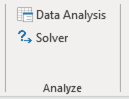From the list of tools it provides, choose Random Number Generation, as shown below, then click OK.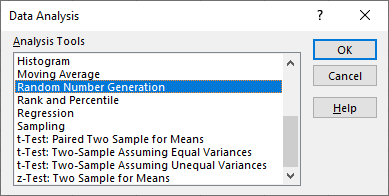Choose a number of variables (that is, columns of output) and of random numbers (that is, rows of output) and a distribution. Once you select a distribution, you can also select its parameters (e.g., the mean and standard deviation for a normal distribution). Choose where you want the output and then click OK.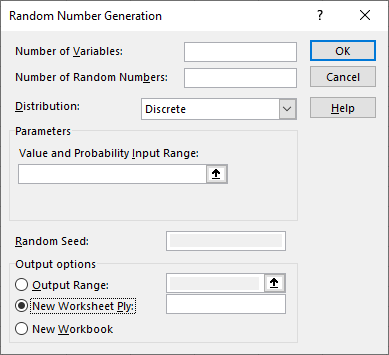Here is example data generated for 3 variables, 20 random numbers per variable, using a standard normal distribution.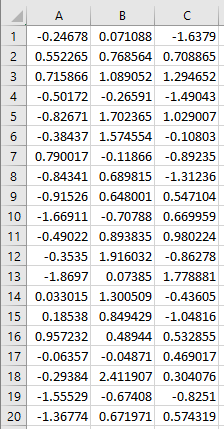It’s also possible to generate random numbers using Excel formulas in place of the Data Analysis Toolpak. Here’s how:

No matter what distribution you want to draw from, begin by generating random values from the uniform distribution on the interval [0,1] using the =RAND() function. For example, if you’ll want 10 random values, place the =RAND() formula into 10 cells in a single column, like so: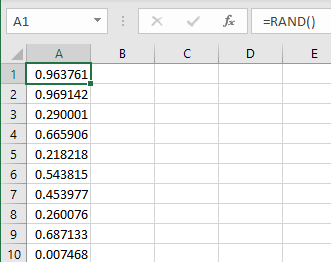Then in an adjacent column, apply one of the built-in inverse CDF functions from Excel’s statistics function set. For example, to generate values from a normal distribution with mean 5 and standard deviation 2, apply =NORM.INV(_,5,2) to each random number in the first column. The NORM.INV function converts uniform random values into random values chosen from the specified distribution.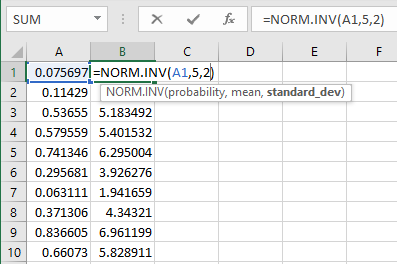Excel also has built-in functions for several other distributions, including BETA.INV, BINOM.INV, CHISQ.INV, F.INV, GAMMA.INV, LOGNORM.INV, and T.INV.

Excel recomputes random values every time a formula or cell changes. If you do not want this behavior, simply copy all the random cells and then paste them back into the exact same locations, but using the “Paste Values” functionality of Excel, which removes the original formulas, leaving only their final results.